## 1. Introduction

Radial breathing mode (RBM) of carbon nanotubes (CNTs) is a low frequency mode, but accounts for the strongest feature observed in the CNT Raman spectrum. For the RBM, all of the carbon atoms in a CNT move in the radial direction synchronously, which generates an effect similar to "breathing" [1, 2]. This mode is unique to CNTs, and is not observed in other carbon systems . Resonant Raman measurement of the RBM in CNTs is a standard, straightforward method for precisely determining the diameter of a CNT, distinguishing the CNT chiral-index assignments, or characterizing CNT conglomerates . For CNTs, pressure studies are motivated by the need to investigate mechanical stability, pressure-induced phase transitions (such as vibrational characteristics), and the effects of intertube interactions. In this letter, the RBM frequency of CNTs subjected to axial pressure is studied using an elastic continuum mechanics model. Single-walled carbon nanotubes (SWCNTs) are described as an individual elastic shell and double-walled carbon nanotubes (DWCNTs) are considered as two shells coupled through the van der Waals (vdW) force interaction between them. The interaction of the vdW force between the inner and outer tubes and the effect of axial pressure are incorporated into the formulation. We consider the effects of the influences of the axial half-wave number m, circumference wave number n, nanotube diameter, and the aspect ratio L/D of the nanotubes on the RBM frequency for SWCNTs and DWCNTs exposed to varying axial pressures. Through comparison with previous results obtained from experiments and simulations, it can be seen that the continuum shell model can be used to predict the RBM frequency of CNTs exposed to various axial pressures.

## 2. Theoretical approach

### 2.1 Governing equations of SWCNTs under axial pressure

A continuum elastic shell model (Figure 1) is used to analyze the characteristics of the RBM frequency of CNTs subjected to varying axial pressures. The cylindrical shell is used to designate a coordinate system (x, θ, z). The coordinates x, θ, and z refer to the axial, circumferential and radial directions, respectively. The displacements for the CNTs are u, v and w corresponding to the x, θ, and z directions, respectively. The dimensions of the nanotubes are defined as the thickness h, radius R, length L, Poisson's ratio v and density ρ.

Based on our previous work  and Love's first approximation shell theory , the equation of motion for simple supported CNTs is given by: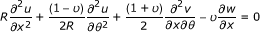(1)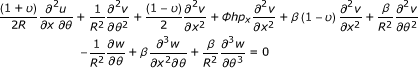(2)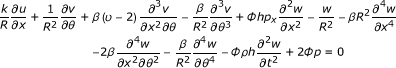(3)

where Φ = (1-v 2)/Eh and β = h 2/12R 2; p x is the axial pressure acting on the both ends of the CNT; and p is the vdW interaction pressure between inner tube and outer tube in a DWCNT, which is shown schematically in Figure 2.

### 2.2 van der Waals interaction forces

To study the vibrational behavior of DWCNTs, a double-elastic shell model was developed that assumes each of the nested tubes in a CNT is an individual elastic shell, and the adjacent tubes are coupled to each other by normal vdW interactions. The pressures exerted on the inner and outer nanotubes through the vdW interaction forces (Figure 2) are given as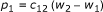(4)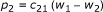(5)

w k (k = 1, 2) are the radial displacements of the inner and outer nanotubes, and c ij (i,j = 1, 2) is the vdW interaction coefficient between nanotubes, which can be estimated using the Lennard-Jones potential :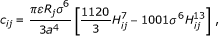(6)

Where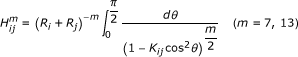(7)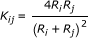(8)

where a is the carbon-carbon bond length (0.142 nm); R i and R j are the inner and outer radii of the DWCNTs; and σ and ε are the vdW radius and the well depth of the Lennard-Jones potential, respectively. The vdW parameters of the DWCNTs in the Lennard-Jones potential are ε = 2.967 meV and σ = 0.34 nm (from Saito et al. ).

### 2.3 RBM frequency of DWCNTs

To model the vdW force, we substitute Eqs. (4) and (5) into Eqs. (1)-(3). The governing equations for the RBM frequency of inner and outer tubes of DWCNTs subjected to an axial pressure can be expressed as:

For inner tube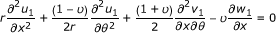(9)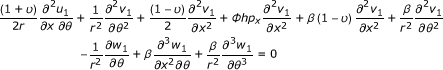(10)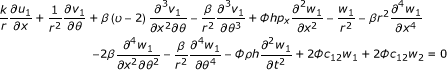(11)

For outer tube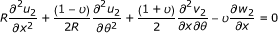(12)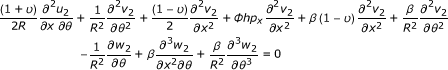(13)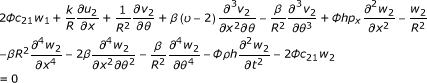(14)

where r and R are the radius of inner tube and outer tube of the DWCNT, respectively. To simplify the calculation, Eqs. (9)-(14) can be rewritten as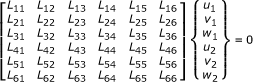(15)

where L ij are the differential operators given as: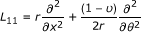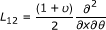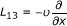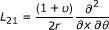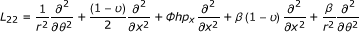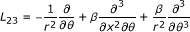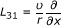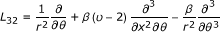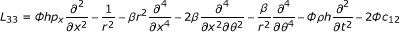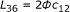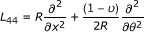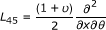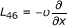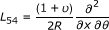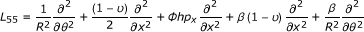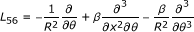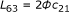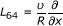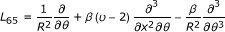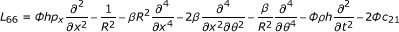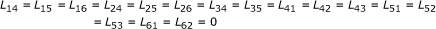(16)

The general solution for the displacements u k , v k and w k in the inner and outer tubes of a DWCNT can be given by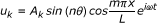(17)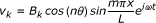(18)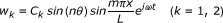(19)

where A k , B k and C k are the longitudinal, circumferential and radial amplitudes of the displacements in the inner (k = 1) and outer tubes (k = 2), respectively. L is the length of CNT which is shown in Figure 1. The wave numbers m and n are the axial half-wave and circumferential numbers, respectively.

## 3. Numerical results and discussion

For the present analysis, an individual SWCNT was assumed to be a graphene sheet rolled into a cylinder and the DWCNT is considered to be two layered nanotube shells coupled by vdW interactions. The value of the thickness of sheet is 0.34 nm; the elastic modulus is 1.0 TPa; the Poisson's ratio is 0.27; the mass density of the CNTs is 2.3 g/cm3; and the inner and outer diameters of the DWCNTs are 2.2 nm and 3.0 nm, respectively .

Based on our proposed theoretical approach, we first calculate the RBM frequency of an isolated SWCNT subjected to zero pressure varying with radius. Note that the commonly used unit for the frequency of the RBM f is in cm-1 for Raman spectroscopy experiments. However, the unit Hertz (Hz) for ω has been adopted for convenience in this study. Our results show that when the diameter is increased from 1.43 to 1.59 nm, the RBM frequency of a SWCNT monotonically decreases from 33.03 to 26.76 THz. To extract the correct parameters for the elastic continuum model, we compared the present work with other approaches. According to Raman scattering technique by Jorio et al. , the frequency of a RBM ranges from 33.18 to 28.46 THz for SWCNTs with diameters of 1.43 to 1.65 nm when structural factors are considered. Furthermore, Peica et al.  used tip-enhanced Raman spectroscopy (TERS) to show that with a change in diameter from 1.35 to 1.63 nm, the RBM frequency changes from 33.44 to 28.29 THz for SWCNTs. Kurti et al.  used first-principles calculations to show that the frequency of the RBMs were between 32.72 and 28.14 THz for SWCNTs with diameters ranging from 1.35 to 1.56 nm. Batra et al.  also investigated the RBM frequency of CNTs using the molecular dynamics (MD) method and finite element method (FEM). They found that the RBM frequency ranged from 31.55 (31.22 for FEM) to 27.40 (27.26) THz for SWCNTs with diameters between 1.35 to 1.56 nm. Our calculated frequencies of the RBM of SWCNTs with varying diameters not exposed to axial pressure agree closely with these previously reported values. This verifies that the continuum elastic shell model can accurately describe the RBM frequency of CNTs.

The RBM frequency ratios of SWCNTs with different axial half-wave numbers subjected to axial pressure in shown in Figure 3. The frequency ratio η is defined as: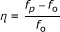(20)

where f p is the frequency of the RBM with exposed to axial pressure, and f 0 is the frequency of RBM not exposed to axial pressure. Note that the RBM frequency ratio η is sublinear with respect to increasing axial pressure. We also found that the axial half-wave number m plays a critical role in this increasing frequency ratio. When the axial half-wave number increases, the increment of change in the speed of the frequency ratio becomes much smaller. The results from nonlinear stick-spiral model, presented by Chang et al. , are also shown for comparison. The models show good agreement when the axial half-wave number m is 1. These results confirm that the largest contribution to the RBM for a SWCNT comes when m = 1. By contrast, m ≥ 2 wave numbers are in the minority.

Figure 4 shows the RBM frequencies of SWCNTs as a function of the aspect ratio L/D (D is the diameter of the SWCNT). The frequencies of the SWCNTs grow sublinear logarithmically with an increasing aspect ratio. When the circumferential wave n is 1, the frequency of the RBM decreases dramatically with increasing aspect ratio. This is especially true for larger aspect ratios, and the variational trend of the RBM frequency is quite different for n = 2, 3, 4 or higher. This is caused by the misalignment of the tubes for the circumferential wave n = 1, which makes the entire SWCNTs unstable. The unstable SWCNTs with n = 1 can be affected by external factors more easily than those with other circumferential waves numbers. This phenomena is unique to SWCNTs with n = 1, and cannot be observed with other circumferential waves numbers. However, the RBM frequency is largely unaffected by the aspect ratio of the tube when n ≥ 2.